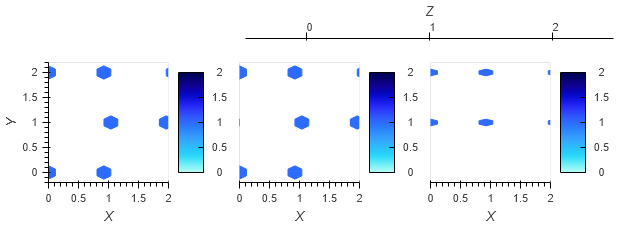# Consistent hexbin hex size

Hi,

I am trying to create a set of hexbin plots with multiple axes using `hvplot`. However, the maximum values are different for different plots. The result is that different plots have different size hexes. This makes direct comparison difficult. Is there a way to have a fixed hex size across all plots? The “gridsize” option doesn’t work, it makes them bigger or smaller but still inconsistent.

Here is an example:

``````import numpy as np
import hvplot.pandas
import xarray as xr

data = np.tri(3, 3, 1).T[..., None]*np.tri(3, 3, 1)
data[data==0] = np.NaN
da = xr.DataArray(data, dims=["X", "Y", "Z"])
da.to_dataframe(name='test').dropna().hvplot.hexbin(x="X", y="Y", row="Z", gridsize=10)
``````

Attached is the result. Notice the hexes are squashed vertically in the third example. Leaving `gridsize` off still has the same problem, but it is less obvious due to their small size.